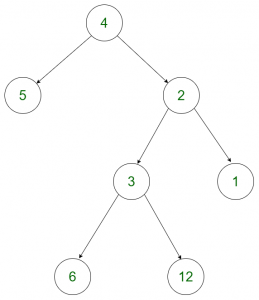Related Articles

# Find the maximum GCD of the siblings of a Binary Tree

• Difficulty Level : Medium
• Last Updated : 28 May, 2021

Given a 2d-array arr[][] which represents the nodes of a Binary tree, the task is to find the maximum GCD of the siblings of this tree without actually constructing it.

Example:

Attention reader! Don’t stop learning now. Get hold of all the important DSA concepts with the DSA Self Paced Course at a student-friendly price and become industry ready.  To complete your preparation from learning a language to DS Algo and many more,  please refer Complete Interview Preparation Course.

In case you wish to attend live classes with experts, please refer DSA Live Classes for Working Professionals and Competitive Programming Live for Students.

Input: arr[][] = {{4, 5}, {4, 2}, {2, 3}, {2, 1}, {3, 6}, {3, 12}}
Output:
Explanation:For the above tree, the maximum GCD for the sibilings is formed for the nodes 6 and 12 for the children of node 3.

Input: arr[][] = {{5, 4}, {5, 8}, {4, 6}, {4, 9}, {8, 10}, {10, 20}, {10, 30}}
Output: 10

Approach: The idea is to form a vector and store the tree in the form of the vector. After storing the tree in the form of a vector, the following cases occur:

• If the vector size is 0 or 1, then print 0 as GCD could not be found.
• For all other cases, since we store the tree in the form of a pair, we consider the first values of two pairs and compare them. But first, you need to Sort the pair of edges.
For example, let’s assume there are two pairs in the vector A and B. We check if:
`A.first == B.first`
• If both of them match, then both of them belongs to the same parent. Therefore, we compute the GCD of the second values in the pairs and finally print the maximum of all such GCD’s.

Below is the implementation of the above approach:

## C++

 `// C++ program to find the maximum``// GCD of the siblings of a binary tree` `#include ``using` `namespace` `std;` `// Function to find maximum GCD``int` `max_gcd(vector >& v)``{``    ``// No child or Single child``    ``if` `(v.size() == 1 || v.size() == 0)``        ``return` `0;``  ` `    ``sort(v.begin(), v.end());` `    ``// To get the first pair``    ``pair<``int``, ``int``> a = v;``    ``pair<``int``, ``int``> b;``    ``int` `ans = INT_MIN;``    ``for` `(``int` `i = 1; i < v.size(); i++) {``        ``b = v[i];` `        ``// If both the pairs belongs to``        ``// the same parent``        ``if` `(b.first == a.first)` `            ``// Update ans with the max``            ``// of current gcd and``            ``// gcd of both children``            ``ans``                ``= max(ans,``                      ``__gcd(a.second,``                            ``b.second));` `        ``// Update previous``        ``// for next iteration``        ``a = b;``    ``}``    ``return` `ans;``}` `// Driver function``int` `main()``{``    ``vector > v;``    ``v.push_back(make_pair(5, 4));``    ``v.push_back(make_pair(5, 8));``    ``v.push_back(make_pair(4, 6));``    ``v.push_back(make_pair(4, 9));``    ``v.push_back(make_pair(8, 10));``    ``v.push_back(make_pair(10, 20));``    ``v.push_back(make_pair(10, 30));` `    ``cout << max_gcd(v);``    ``return` `0;``}`

## Java

 `// Java program to find the maximum``// GCD of the siblings of a binary tree``import` `java.util.*;``import` `java.lang.*;` `class` `GFG{``    ` `// Function to find maximum GCD``static` `int` `max_gcd(ArrayList<``int``[]> v)``{``    ` `    ``// No child or Single child``    ``if` `(v.size() == ``1` `|| v.size() == ``0``)``        ``return` `0``;``  ` `      ``Collections.sort(v, ``new` `Comparator<``int``[]>() {``        ``public` `int` `compare(``int``[] a, ``int``[] b) {``            ``return` `a[``0``]-b[``0``];``        ``}``    ``});``  ` `    ``// To get the first pair``    ``int``[] a = v.get(``0``);``    ``int``[] b = ``new` `int``[``2``];``    ``int` `ans = Integer.MIN_VALUE;``    ` `    ``for``(``int` `i = ``1``; i < v.size(); i++)``    ``{``        ``b = v.get(i);``        ` `        ``// If both the pairs belongs to``        ``// the same parent``        ``if` `(b[``0``] == a[``0``])``  ` `            ``// Update ans with the max``            ``// of current gcd and``            ``// gcd of both children``            ``ans = Math.max(ans,``                           ``gcd(a[``1``],``                               ``b[``1``]));``      ` `        ``// Update previous``        ``// for next iteration``        ``a = b;``    ``}``    ``return` `ans;``} ` `static` `int` `gcd(``int` `a, ``int` `b)``{``    ``if` `(b == ``0``)``        ``return` `a;``        ` `    ``return` `gcd(b, a % b);``}` `// Driver code``public` `static` `void` `main(String[] args)``{``    ``ArrayList<``int``[]> v = ``new` `ArrayList<>();``    ` `    ``v.add(``new` `int``[]{``5``, ``4``});``    ``v.add(``new` `int``[]{``5``, ``8``});``    ``v.add(``new` `int``[]{``4``, ``6``});``    ``v.add(``new` `int``[]{``4``, ``9``});``    ``v.add(``new` `int``[]{``8``, ``10``});``    ``v.add(``new` `int``[]{``10``, ``20``});``    ``v.add(``new` `int``[]{``10``, ``30``});``    ` `    ``System.out.println(max_gcd(v));``}``}` `// This code is contributed by offbeat`

## Python3

 `# Python3 program to find the maximum``# GCD of the siblings of a binary tree``from` `math ``import` `gcd` `# Function to find maximum GCD``def` `max_gcd(v):` `    ``# No child or Single child``    ``if` `(``len``(v) ``=``=` `1` `or` `len``(v) ``=``=` `0``):``        ``return` `0``      ` `    ``v.sort()` `    ``# To get the first pair``    ``a ``=` `v[``0``]``    ``ans ``=` `-``10``*``*``9``    ``for` `i ``in` `range``(``1``, ``len``(v)):``        ``b ``=` `v[i]` `        ``# If both the pairs belongs to``        ``# the same parent``        ``if` `(b[``0``] ``=``=` `a[``0``]):` `            ``# Update ans with the max``            ``# of current gcd and``            ``# gcd of both children``            ``ans ``=` `max``(ans, gcd(a[``1``], b[``1``]))` `        ``# Update previous``        ``# for next iteration``        ``a ``=` `b``    ``return` `ans` `# Driver function``if` `__name__ ``=``=` `'__main__'``:``    ``v``=``[]``    ``v.append([``5``, ``4``])``    ``v.append([``5``, ``8``])``    ``v.append([``4``, ``6``])``    ``v.append([``4``, ``9``])``    ``v.append([``8``, ``10``])``    ``v.append([``10``, ``20``])``    ``v.append([``10``, ``30``])` `    ``print``(max_gcd(v))` `# This code is contributed by mohit kumar 29   `

## C#

 `// C# program to find the maximum``// GCD of the siblings of a binary tree``using` `System.Collections;``using` `System;` `class` `GFG{``     ` `// Function to find maximum GCD``static` `int` `max_gcd(ArrayList v)``{``     ` `    ``// No child or Single child``    ``if` `(v.Count == 1 || v.Count == 0)``        ``return` `0;``  ` `    ``v.Sort();``   ` `    ``// To get the first pair``    ``int``[] a = (``int` `[])v;``    ``int``[] b = ``new` `int``;``    ``int` `ans = -10000000;``     ` `    ``for``(``int` `i = 1; i < v.Count; i++)``    ``{``      ``b = (``int``[])v[i];``      ` `      ``// If both the pairs belongs to``      ``// the same parent``      ``if` `(b == a)``        ` `        ``// Update ans with the max``        ``// of current gcd and``        ``// gcd of both children``        ``ans = Math.Max(ans, gcd(a, b));``      ` `      ``// Update previous``      ``// for next iteration``      ``a = b;``    ``}``    ``return` `ans;``} `` ` `static` `int` `gcd(``int` `a, ``int` `b)``{``    ``if` `(b == 0)``        ``return` `a;``         ` `    ``return` `gcd(b, a % b);``}`` ` `// Driver code``public` `static` `void` `Main(``string``[] args)``{``    ``ArrayList v = ``new` `ArrayList();``     ` `    ``v.Add(``new` `int``[]{5, 4});``    ``v.Add(``new` `int``[]{5, 8});``    ``v.Add(``new` `int``[]{4, 6});``    ``v.Add(``new` `int``[]{4, 9});``    ``v.Add(``new` `int``[]{8, 10});``    ``v.Add(``new` `int``[]{10, 20});``    ``v.Add(``new` `int``[]{10, 30});``     ` `    ``Console.Write(max_gcd(v));``}``}` `// This code is contributed by rutvik_56`

## Javascript

 ``
Output:
`10`

My Personal Notes arrow_drop_up